library(dplyr)
library(qpNCA)
library(knitr)

Define mutate_cond and locf functions

mutate_cond <- function (.data, condition, ..., envir = parent.frame()){
condition <- eval(substitute(condition), .data, envir)
if(!any(condition))return(.data) # do nothing if nothing to do
.data[condition, ] <- .data[condition, ] %>% mutate(...)
.data
}
locf <- function(x){
good <- !is.na(x)
positions <- seq(length(x))
good.positions <- good * positions
last.good.position <- cummax(good.positions)
last.good.position[last.good.position == 0] <- NA
x[last.good.position]
}

Check out Theoph data and prepare data for NCA

We use the internal Theoph dataset as input file, modify the data and add necessary columns
Furthermore, we introduce some missing values, LOQ values and time deviations

Subject Wt Dose Time conc
1 79.6 4.02 0.00 0.74
1 79.6 4.02 0.25 2.84
1 79.6 4.02 0.57 6.57
1 79.6 4.02 1.12 10.50
1 79.6 4.02 2.02 9.66
1 79.6 4.02 3.82 8.58
input.data <- Theoph

#we need nominal time variable for some tasks.

input.data %<>%
group_by(Subject) %>%
mutate(subject=as.numeric(Subject),
rn=row_number(),
dose=Dose*Wt,
bloq=ifelse(conc==0,1,0),
loq=0.1,
excl_th=0
) %>%
ungroup %>%
## Joining, by = "rn"
input.data %<>%

Impute LOQ values

loqed.data = input.data %>%
correct.loq(
by = "subject",
depvar = "conc",
bloqvar = "bloq",
loqvar = "loq",
loqrule = 1
)

# Result:

loqed.data %>% filter(loqrule.nr!="") %>% select(subject,ntad,conc,loqrule.nr,loqrule.txt) %>% kable()
1 0 0 LOQ1 BLOQ values before first measurable concentration set to 0
3 0 0 LOQ1 BLOQ values before first measurable concentration set to 0
4 0 0 LOQ1 BLOQ values before first measurable concentration set to 0
5 0 0 LOQ1 BLOQ values before first measurable concentration set to 0
6 0 0 LOQ1 BLOQ values before first measurable concentration set to 0
6 24 NA LOQ1 BLOQ values after first measurable concentration set to missing
7 0 0 LOQ1 BLOQ values before first measurable concentration set to 0
8 0 0 LOQ1 BLOQ values before first measurable concentration set to 0
9 0 0 LOQ1 BLOQ values before first measurable concentration set to 0
12 0 0 LOQ1 BLOQ values before first measurable concentration set to 0

Estimation of terminal half-life

th = loqed.data %>%
est.thalf(
by = "subject",
depvar = "conc",
includeCmax = "Y",
exclvar = "excl_th"
)

# Result:

subject no.points intercept lambda_z r.squared adj.r.squared start_th end_th thalf includeCmax points_excluded
1 3 8.212078 0.0915758 0.9989638 0.9979276 9.22 23.85 7.569107 Y N
2 3 8.959057 0.0777431 0.9964163 0.9928325 6.98 12.05 8.915865 Y N
3 6 8.688001 0.0818231 0.9962482 0.9953103 2.02 24.12 8.471285 Y Y
4 3 8.703249 0.0962171 0.9999286 0.9998572 7.03 24.08 7.203989 Y N
5 5 10.568234 0.0948933 0.9957650 0.9943534 3.62 24.17 7.304489 Y N
6 3 12.790536 0.1192526 0.9867217 0.9734435 7.03 12.00 5.812428 Y N

Plot individual regression plots

plot_reg(
loqed.data,
by = "subject",
th = th,
bloqvar = "bloq",
depvar = "conc",
timelab = "Time (h)",
deplab = "Conc (ng/mL)",
exclvar = "excl_th",
plotdir = NA
)
## []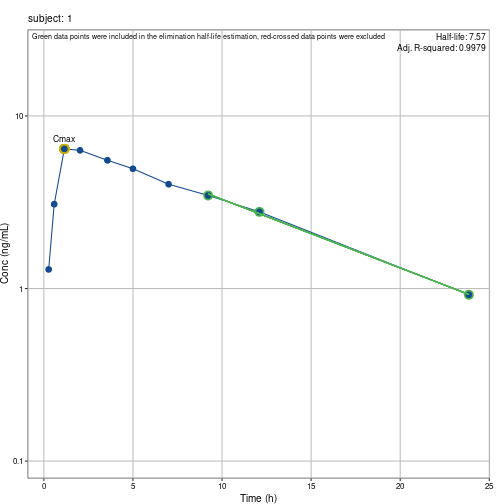##
## []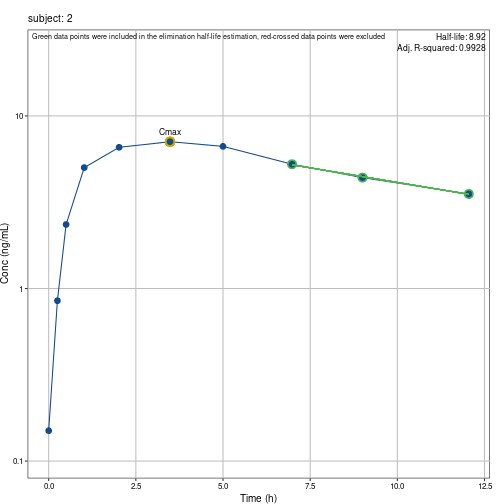##
## []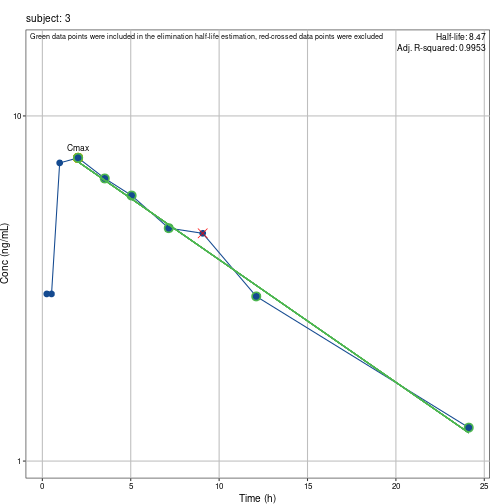##
## []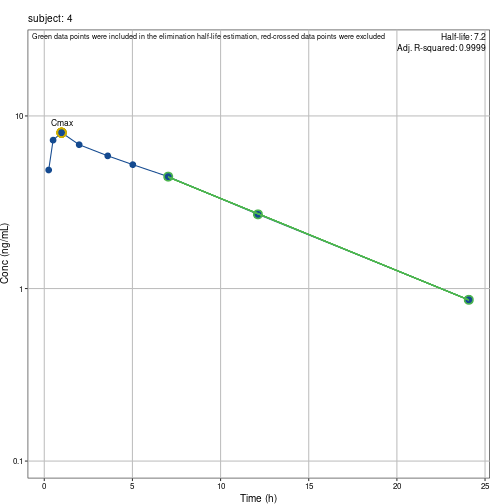##
## []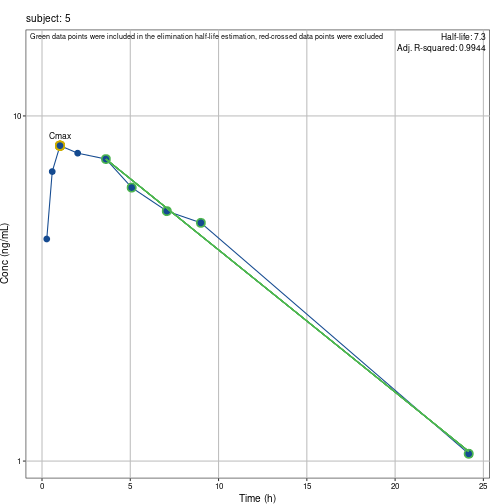##
## []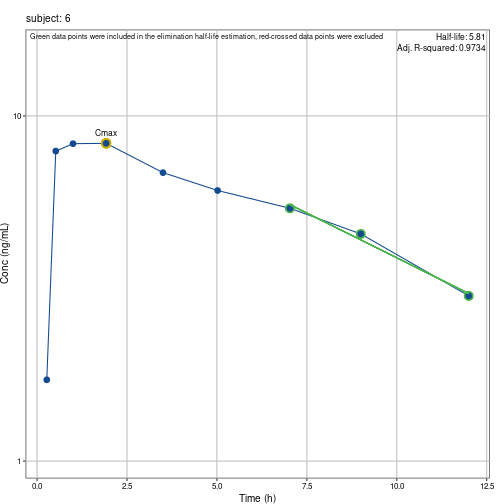##
## []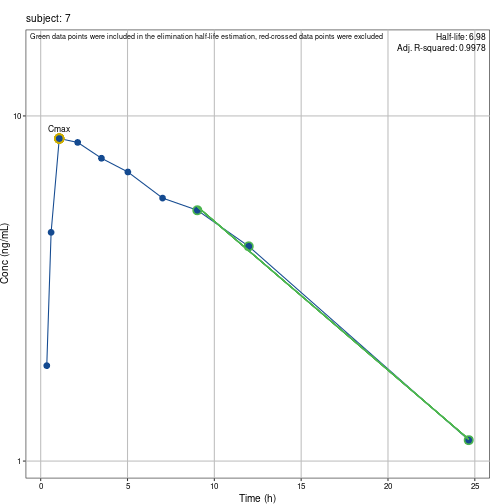##
## []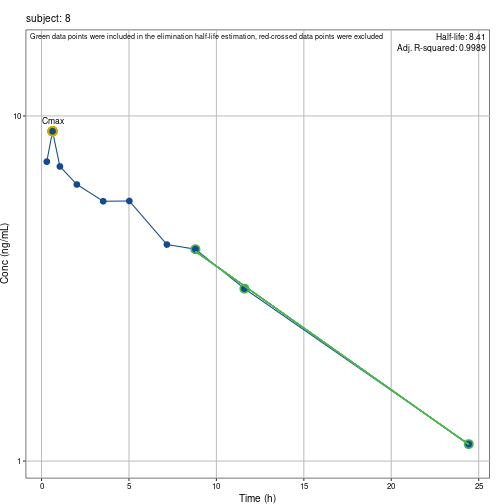##
## []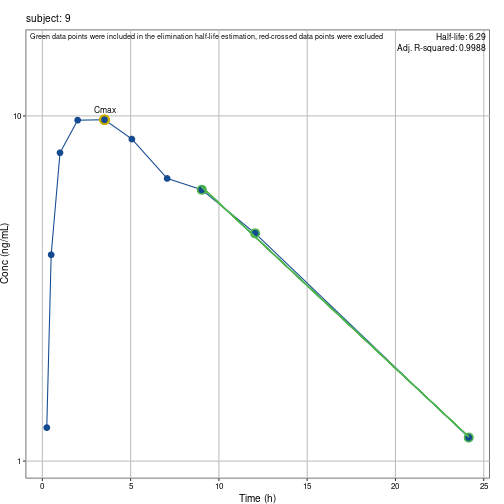##
## []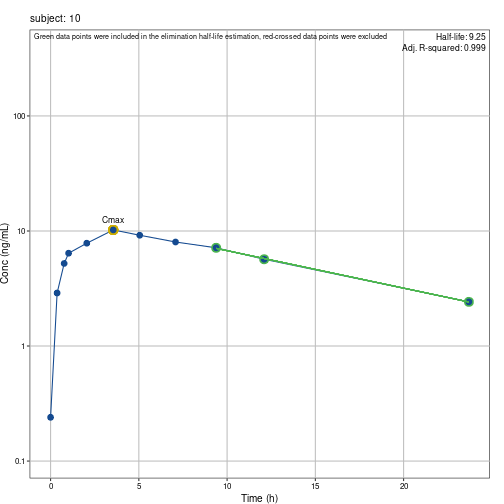##
## []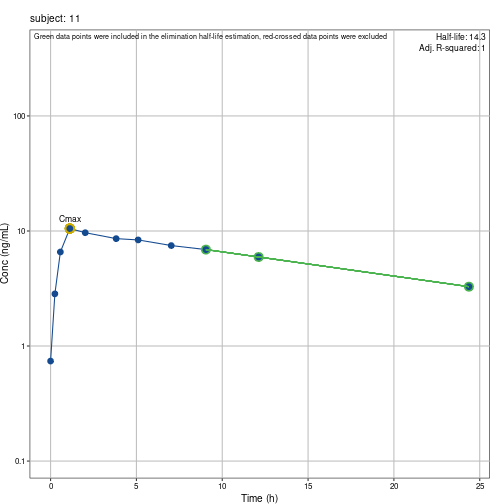##
## []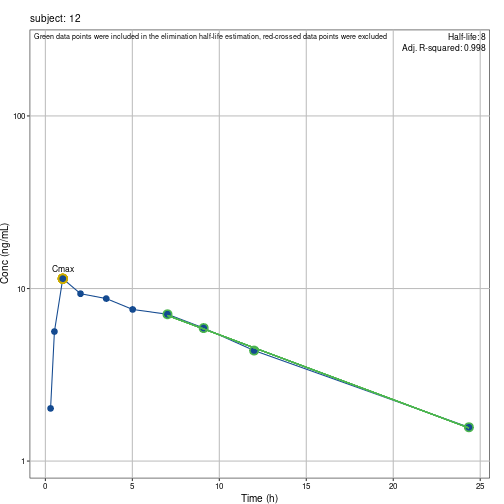Calculate Cmax and Tmax

ctmax = input.data %>% calc.ctmax(
by = "subject",
depvar="conc"
)

# Result:

subject cmax tmax
1 6.44 1.15
2 7.09 3.48
3 7.56 2.02
4 8.00 0.98
5 8.20 1.02
6 8.33 1.92

Correct time deviations at critical time points

ct.data= loqed.data %>%
correct.time(
by="subject",
depvar="conc",
th=th,
tau=24,
tstart=4,
tend=9,
teval=12,
reg="sd",
method=1
)
## Joining, by = "ptime"
## Joining, by = "ptime"
## Joining, by = "ptime"
## Joining, by = "ptime"
## Joining, by = "ptime"
## Joining, by = "ptime"
## Joining, by = "ptime"
## Joining, by = "ptime"
## Joining, by = "ptime"
## Joining, by = "ptime"
## Joining, by = "ptime"
## Joining, by = "ptime"
# Result:

ct.data %>% filter(subject<=5&(trule.nr!=""|create.nr!="")) %>% select(subject,ntad,conc,applies.to.time,trule.nr,trule.txt,create.txt) %>% kable()
subject ntad conc applies.to.time trule.nr trule.txt create.txt
1 4 5.53 TSTART SDT-2 Concentration ( 5.53 ) at deviating time ( t=3.57 instead of 4 ) corrected to 5.353 by interpolation (sample taken too early)
1 9 3.46 TEND SDT-2 Concentration ( 3.46 ) at deviating time ( t=9.22 instead of 9 ) corrected to 3.515 by interpolation (sample taken too late)
1 12 2.78 TEVAL SDT-2 Concentration ( 2.78 ) at deviating time ( t=12.1 instead of 12 ) corrected to 2.804 by interpolation (sample taken too late)
1 24 0.92 TAU SDT-3 Concentration ( 0.92 ) at deviating time ( t=23.85 instead of 24 ) corrected to 0.907 by extrapolation (sample taken too early)
2 4 7.09 TSTART SDT-2 Concentration ( 7.09 ) at deviating time ( t=3.48 instead of 4 ) corrected to 6.943 by interpolation (sample taken too early)
2 12 3.53 TEVAL SDT-2 Concentration ( 3.53 ) at deviating time ( t=12.05 instead of 12 ) corrected to 3.544 by interpolation (sample taken too late)
2 24 NA TAU SDT-2 Concentration ( NA ) at deviating time ( t=24.22 instead of 24 ) corrected to NA by interpolation (sample taken too late)
3 4 6.59 TSTART SDT-2 Concentration ( 6.59 ) at deviating time ( t=3.53 instead of 4 ) corrected to 6.37 by interpolation (sample taken too early)
3 9 4.57 TEND SDT-2 Concentration ( 4.57 ) at deviating time ( t=9.07 instead of 9 ) corrected to 4.576 by interpolation (sample taken too late)
3 12 3.00 TEVAL SDT-2 Concentration ( 3 ) at deviating time ( t=12.1 instead of 12 ) corrected to 3.052 by interpolation (sample taken too late)
3 24 1.25 TAU SDT-2 Concentration ( 1.25 ) at deviating time ( t=24.12 instead of 24 ) corrected to 1.267 by interpolation (sample taken too late)
4 4 5.87 TSTART SDT-2 Concentration ( 5.87 ) at deviating time ( t=3.6 instead of 4 ) corrected to 5.687 by interpolation (sample taken too early)
4 9 NA TEND SDT-2 Concentration ( NA ) at deviating time ( t=9.03 instead of 9 ) corrected to NA by interpolation (sample taken too late)
4 12 2.69 TEVAL SDT-2 Concentration ( 2.69 ) at deviating time ( t=12.12 instead of 12 ) corrected to 2.731 by interpolation (sample taken too late)
4 24 0.86 TAU SDT-2 Concentration ( 0.86 ) at deviating time ( t=24.08 instead of 24 ) corrected to 0.872 by interpolation (sample taken too late)
5 4 7.50 TSTART SDT-2 Concentration ( 7.5 ) at deviating time ( t=3.62 instead of 4 ) corrected to 7.162 by interpolation (sample taken too early)
5 12 NA Missing record at t=12 added
5 24 1.05 TAU SDT-2 Concentration ( 1.05 ) at deviating time ( t=24.17 instead of 24 ) corrected to 1.093 by interpolation (sample taken too late)

Impute missing concentrations at critical time points

cc.ct.data = ct.data %>%
correct.conc(
by ="subject",
tau=24,
tstart=4,
tend=9,
teval=12,
th=th,
reg="sd",
ss="n",
route="EV",
method=1
)
## Joining, by = "ptime"
## Joining, by = "ptime"
## Joining, by = "ptime"
## Joining, by = "ptime"
## Joining, by = "ptime"
## Joining, by = "ptime"
## Joining, by = "ptime"
## Joining, by = "ptime"
## Joining, by = "ptime"
## Joining, by = "ptime"
## Joining, by = "ptime"
## Joining, by = "ptime"
## Joining, by = "ptime"
## Joining, by = "ptime"
## Joining, by = "ptime"
## Joining, by = "ptime"
## Joining, by = "ptime"
## Joining, by = "ptime"
## Joining, by = "ptime"
## Joining, by = "ptime"
## Joining, by = "ptime"
## Joining, by = "ptime"
## Joining, by = "ptime"
## Joining, by = "ptime"
## Joining, by = "ptime"
## Joining, by = "ptime"
## Joining, by = "ptime"
## Joining, by = "ptime"
## Joining, by = "ptime"
## Joining, by = "ptime"
## Joining, by = "ptime"
## Joining, by = "ptime"
## Joining, by = "ptime"
## Joining, by = "ptime"
## Joining, by = "ptime"
## Joining, by = "ptime"
# Result:

cc.ct.data %>% filter(crule.nr!="") %>% select(subject,ntad,conc,applies.to.conc,crule.nr,crule.txt) %>% kable()
subject ntad conc applies.to.conc crule.nr crule.txt
2 0 0.15 PREDOSE SDC-1 Missing or measurable concentration at (SD) PREDOSE set to 0
2 24 NA TAU SDC-3 Missing concentration at t=24 corrected to 1.394 by extrapolation
4 9 NA TEND SDC-2 Missing concentration at t=9 corrected to 3.769 by interpolation
5 12 NA TEVAL SDC-2 Missing concentration at t=12 corrected to 4.139 by interpolation
6 24 NA TAU SDC-3 Missing concentration at t=24 corrected to 0.72 by extrapolation
10 0 0.24 PREDOSE SDC-1 Missing or measurable concentration at (SD) PREDOSE set to 0
11 0 0.74 PREDOSE SDC-1 Missing or measurable concentration at (SD) PREDOSE set to 0

Tabulate corrections

tab_corr = cc.ct.data %>%
tab.corr(
by="subject",
)

# Result:

tab_corr %>% kable()
1 0 NA LOQ1 BLOQ values before first measurable concentration set to 0
1 4 TSTART SDT-2 Concentration ( 5.53 ) at deviating time ( t=3.57 instead of 4 ) corrected to 5.353 by interpolation (sample taken too early)
1 9 TEND SDT-2 Concentration ( 3.46 ) at deviating time ( t=9.22 instead of 9 ) corrected to 3.515 by interpolation (sample taken too late)
1 12 TEVAL SDT-2 Concentration ( 2.78 ) at deviating time ( t=12.1 instead of 12 ) corrected to 2.804 by interpolation (sample taken too late)
1 24 TAU SDT-3 Concentration ( 0.92 ) at deviating time ( t=23.85 instead of 24 ) corrected to 0.907 by extrapolation (sample taken too early)
2 0 PREDOSE SDC-1 Missing or measurable concentration at (SD) PREDOSE set to 0
2 4 TSTART SDT-2 Concentration ( 7.09 ) at deviating time ( t=3.48 instead of 4 ) corrected to 6.943 by interpolation (sample taken too early)
2 12 TEVAL SDT-2 Concentration ( 3.53 ) at deviating time ( t=12.05 instead of 12 ) corrected to 3.544 by interpolation (sample taken too late)
2 24 TAU SDT-2 Concentration ( NA ) at deviating time ( t=24.22 instead of 24 ) corrected to NA by interpolation (sample taken too late)
2 24 TAU SDC-3 Missing concentration at t=24 corrected to 1.394 by extrapolation
3 0 NA LOQ1 BLOQ values before first measurable concentration set to 0
3 4 TSTART SDT-2 Concentration ( 6.59 ) at deviating time ( t=3.53 instead of 4 ) corrected to 6.37 by interpolation (sample taken too early)
3 9 TEND SDT-2 Concentration ( 4.57 ) at deviating time ( t=9.07 instead of 9 ) corrected to 4.576 by interpolation (sample taken too late)
3 12 TEVAL SDT-2 Concentration ( 3 ) at deviating time ( t=12.1 instead of 12 ) corrected to 3.052 by interpolation (sample taken too late)
3 24 TAU SDT-2 Concentration ( 1.25 ) at deviating time ( t=24.12 instead of 24 ) corrected to 1.267 by interpolation (sample taken too late)
4 0 NA LOQ1 BLOQ values before first measurable concentration set to 0
4 4 TSTART SDT-2 Concentration ( 5.87 ) at deviating time ( t=3.6 instead of 4 ) corrected to 5.687 by interpolation (sample taken too early)
4 9 TEND SDT-2 Concentration ( NA ) at deviating time ( t=9.03 instead of 9 ) corrected to NA by interpolation (sample taken too late)
4 9 TEND SDC-2 Missing concentration at t=9 corrected to 3.769 by interpolation
4 12 TEVAL SDT-2 Concentration ( 2.69 ) at deviating time ( t=12.12 instead of 12 ) corrected to 2.731 by interpolation (sample taken too late)
4 24 TAU SDT-2 Concentration ( 0.86 ) at deviating time ( t=24.08 instead of 24 ) corrected to 0.872 by interpolation (sample taken too late)
5 0 NA LOQ1 BLOQ values before first measurable concentration set to 0
5 4 TSTART SDT-2 Concentration ( 7.5 ) at deviating time ( t=3.62 instead of 4 ) corrected to 7.162 by interpolation (sample taken too early)
5 12 NA - Missing record at t=12 added
5 12 TEVAL SDC-2 Missing concentration at t=12 corrected to 4.139 by interpolation
5 24 TAU SDT-2 Concentration ( 1.05 ) at deviating time ( t=24.17 instead of 24 ) corrected to 1.093 by interpolation (sample taken too late)
6 0 NA LOQ1 BLOQ values before first measurable concentration set to 0
6 4 TSTART SDT-2 Concentration ( 6.85 ) at deviating time ( t=3.5 instead of 4 ) corrected to 6.597 by interpolation (sample taken too early)
6 24 NA LOQ1 BLOQ values after first measurable concentration set to missing
6 24 TAU SDT-2 Concentration ( NA ) at deviating time ( t=24.3 instead of 24 ) corrected to NA by interpolation (sample taken too late)
6 24 TAU SDC-3 Missing concentration at t=24 corrected to 0.72 by extrapolation
7 0 NA LOQ1 BLOQ values before first measurable concentration set to 0
7 4 TSTART SDT-2 Concentration ( 7.54 ) at deviating time ( t=3.5 instead of 4 ) corrected to 7.323 by interpolation (sample taken too early)
7 9 TEND SDT-2 Concentration ( 5.33 ) at deviating time ( t=9.02 instead of 9 ) corrected to 5.335 by interpolation (sample taken too late)
7 12 TEVAL SDT-2 Concentration ( 4.19 ) at deviating time ( t=11.98 instead of 12 ) corrected to 4.185 by interpolation (sample taken too early)
7 24 TAU SDT-2 Concentration ( 1.15 ) at deviating time ( t=24.65 instead of 24 ) corrected to 1.306 by interpolation (sample taken too late)
8 0 NA LOQ1 BLOQ values before first measurable concentration set to 0
8 4 TSTART SDT-2 Concentration ( 5.66 ) at deviating time ( t=3.53 instead of 4 ) corrected to 5.663 by interpolation (sample taken too early)
8 9 TEND SDT-2 Concentration ( 4.11 ) at deviating time ( t=8.8 instead of 9 ) corrected to 4.042 by interpolation (sample taken too early)
8 12 TEVAL SDT-2 Concentration ( 3.16 ) at deviating time ( t=11.6 instead of 12 ) corrected to 3.096 by interpolation (sample taken too early)
8 24 TAU SDT-2 Concentration ( 1.12 ) at deviating time ( t=24.43 instead of 24 ) corrected to 1.188 by interpolation (sample taken too late)
9 0 NA LOQ1 BLOQ values before first measurable concentration set to 0
9 4 TSTART SDT-2 Concentration ( 9.75 ) at deviating time ( t=3.52 instead of 4 ) corrected to 9.385 by interpolation (sample taken too early)
9 9 TEND SDT-2 Concentration ( 6.11 ) at deviating time ( t=9.03 instead of 9 ) corrected to 6.117 by interpolation (sample taken too late)
9 12 TEVAL SDT-2 Concentration ( 4.57 ) at deviating time ( t=12.05 instead of 12 ) corrected to 4.595 by interpolation (sample taken too late)
9 24 TAU SDT-2 Concentration ( 1.17 ) at deviating time ( t=24.15 instead of 24 ) corrected to 1.212 by interpolation (sample taken too late)
10 0 PREDOSE SDC-1 Missing or measurable concentration at (SD) PREDOSE set to 0
10 4 TSTART SDT-2 Concentration ( 10.21 ) at deviating time ( t=3.55 instead of 4 ) corrected to 9.901 by interpolation (sample taken too early)
10 9 TEND SDT-2 Concentration ( 7.14 ) at deviating time ( t=9.38 instead of 9 ) corrected to 7.285 by interpolation (sample taken too late)
10 12 TEVAL SDT-2 Concentration ( 5.68 ) at deviating time ( t=12.1 instead of 12 ) corrected to 5.734 by interpolation (sample taken too late)
10 24 TAU SDT-3 Concentration ( 2.42 ) at deviating time ( t=23.7 instead of 24 ) corrected to 2.366 by extrapolation (sample taken too early)
11 0 PREDOSE SDC-1 Missing or measurable concentration at (SD) PREDOSE set to 0
11 4 TSTART SDT-2 Concentration ( 8.58 ) at deviating time ( t=3.82 instead of 4 ) corrected to 8.549 by interpolation (sample taken too early)
11 9 TEND SDT-2 Concentration ( 6.89 ) at deviating time ( t=9.05 instead of 9 ) corrected to 6.904 by interpolation (sample taken too late)
11 12 TEVAL SDT-2 Concentration ( 5.94 ) at deviating time ( t=12.12 instead of 12 ) corrected to 5.977 by interpolation (sample taken too late)
11 24 TAU SDT-2 Concentration ( 3.28 ) at deviating time ( t=24.37 instead of 24 ) corrected to 3.36 by interpolation (sample taken too late)
12 0 NA LOQ1 BLOQ values before first measurable concentration set to 0
12 4 TSTART SDT-2 Concentration ( 8.74 ) at deviating time ( t=3.5 instead of 4 ) corrected to 8.352 by interpolation (sample taken too early)
12 9 TEND SDT-2 Concentration ( 5.9 ) at deviating time ( t=9.1 instead of 9 ) corrected to 5.957 by interpolation (sample taken too late)
12 24 TAU SDT-2 Concentration ( 1.57 ) at deviating time ( t=24.35 instead of 24 ) corrected to 1.649 by interpolation (sample taken too late)

Calculate PK parameters NOT based on lambda_z

par = cc.ct.data %>%
calc.par(by = 'subject',
tau=24,
teval=12,
tstart=4,
tend=9,
route="EV",
method=1)

# Result:

subject route method tlast clast.obs tlast.ok t0.ok aucall auclast aumcall aumclast mrtall mrtlast calc.tau auctau aumctau tau calc.teval auc12 teval calc.part auc4_9 tstart tend c0 area.back.extr
1 EV 1 23.85 0.92 1 1 73.77555 73.77555 609.1524 609.1524 8.256833 8.256833 1 73.97837 612.3497 24 1 51.75887 12 1 21.64179 4 9 NA NA
2 EV 1 12.05 3.53 1 1 62.25685 62.25685 354.0998 354.0998 5.687724 5.687724 1 91.67850 808.1730 24 1 62.08000 12 1 28.32875 4 9 NA NA
3 EV 1 24.12 1.25 1 1 88.55995 88.55995 739.5346 739.5346 8.350666 8.350666 1 88.40890 737.1499 24 1 62.71486 12 1 26.17989 4 9 NA NA
4 EV 1 24.08 0.86 1 1 80.44595 80.44595 614.9855 614.9855 7.644705 7.644705 1 80.37666 614.1894 24 1 58.89166 12 1 23.37641 4 9 NA NA
5 EV 1 24.17 1.05 1 1 102.32475 102.32475 767.2143 767.2143 7.497837 7.497837 1 102.14258 767.7359 24 1 70.75194 12 1 28.50079 4 9 NA NA
6 EV 1 12.00 3.01 1 1 67.48030 67.48030 349.9481 349.9481 5.185929 5.185929 1 89.85776 670.2872 24 1 67.48030 12 1 27.80327 4 9 NA NA

Calculate PK parameters based on lambda_z

# Create a covariates file, containing at least the dose given

cov = input.data %>%
distinct(subject,dose)

par <- par %>%
calc.par.th(
by="subject",
th=th ,
covariates=cov,
dose="dose",
factor=1,
reg="sd",
ss="n"
)

# Result:

subject method tlast clast.obs tlast.ok t0.ok aucall auclast aumcall aumclast mrtall mrtlast calc.tau auctau aumctau tau calc.teval auc12 teval calc.part auc4_9 tstart tend c0 area.back.extr no.points intercept lambda_z r.squared adj.r.squared start_th end_th thalf includeCmax points_excluded dose factor reg ss route clast.pred aucinf.obs aucinf.pred aumcinf.obs aumcinf.pred cl.f.obs cl.f.pred mrtinf.obs mrtinf.pred vz.f.obs vz.f.pred vss.obs vss.pred pctextr.obs pctextr.pred pctback.obs pctback.pred cl.obs cl.pred vz.obs vz.pred cl.f.ss cl.ss
1 1 23.85 0.92 1 1 73.77555 73.77555 609.1524 609.1524 8.256833 8.256833 1 73.97837 612.3497 24 1 51.75887 12 1 21.64179 4 9 NA NA 3 8.212078 0.0915758 0.9989638 0.9979276 9.22 23.85 7.569107 Y N 320.000 1 sd n ev 0.9245229 83.82187 83.87126 958.4620 960.1793 3.817620 3.815371 11.434510 11.448252 41.68807 41.66352 NA NA 11.985320 12.037150 NA NA NA NA NA NA NA NA
2 1 12.05 3.53 1 1 62.25685 62.25685 354.0998 354.0998 5.687724 5.687724 1 91.67850 808.1730 24 1 62.08000 12 1 28.32875 4 9 NA NA 3 8.959057 0.0777431 0.9964163 0.9928325 6.98 12.05 8.915865 Y N 319.770 1 sd n ev 3.5108576 107.66280 107.41657 1485.2925 1479.1583 2.970107 2.976915 13.795782 13.770299 38.20411 38.29168 NA NA 42.174223 42.041671 NA NA NA NA NA NA NA NA
3 1 24.12 1.25 1 1 88.55995 88.55995 739.5346 739.5346 8.350666 8.350666 1 88.40890 737.1499 24 1 62.71486 12 1 26.17989 4 9 NA NA 6 8.688001 0.0818231 0.9962482 0.9953103 2.02 24.12 8.471285 Y Y 319.365 1 sd n ev 1.2072785 103.83680 103.31468 1294.7180 1275.7434 3.075644 3.091187 12.468778 12.348132 37.58892 37.77888 NA NA 14.712367 14.281349 NA NA NA NA NA NA NA NA
4 1 24.08 0.86 1 1 80.44595 80.44595 614.9855 614.9855 7.644705 7.644705 1 80.37666 614.1894 24 1 58.89166 12 1 23.37641 4 9 NA NA 3 8.703249 0.0962171 0.9999286 0.9998572 7.03 24.08 7.203989 Y N 319.800 1 sd n ev 0.8579476 89.38407 89.36274 923.1107 922.3753 3.577819 3.578673 10.327463 10.321700 37.18484 37.19372 NA NA 9.999676 9.978193 NA NA NA NA NA NA NA NA
5 1 24.17 1.05 1 1 102.32475 102.32475 767.2143 767.2143 7.497837 7.497837 1 102.14258 767.7359 24 1 70.75194 12 1 28.50079 4 9 NA NA 5 10.568234 0.0948933 0.9957650 0.9943534 3.62 24.17 7.304489 Y N 319.365 1 sd n ev 1.0663923 113.38981 113.56255 1151.2620 1157.2576 2.816523 2.812239 10.153135 10.190486 29.68094 29.63579 NA NA 9.758423 9.895693 NA NA NA NA NA NA NA NA
6 1 12.00 3.01 1 1 67.48030 67.48030 349.9481 349.9481 5.185929 5.185929 1 89.85776 670.2872 24 1 67.48030 12 1 27.80327 4 9 NA NA 3 12.790536 0.1192526 0.9867217 0.9734435 7.03 12.00 5.812428 Y N 318.560 1 sd n ev 3.0577347 92.72084 93.12112 864.4906 872.6506 3.435689 3.420921 9.323585 9.371135 28.81018 28.68634 NA NA 27.222078 27.534915 NA NA NA NA NA NA NA NA

Combine All NCA parameters in one data frame

par_all = left_join(par, ctmax)
## Joining, by = "subject"
# Result: